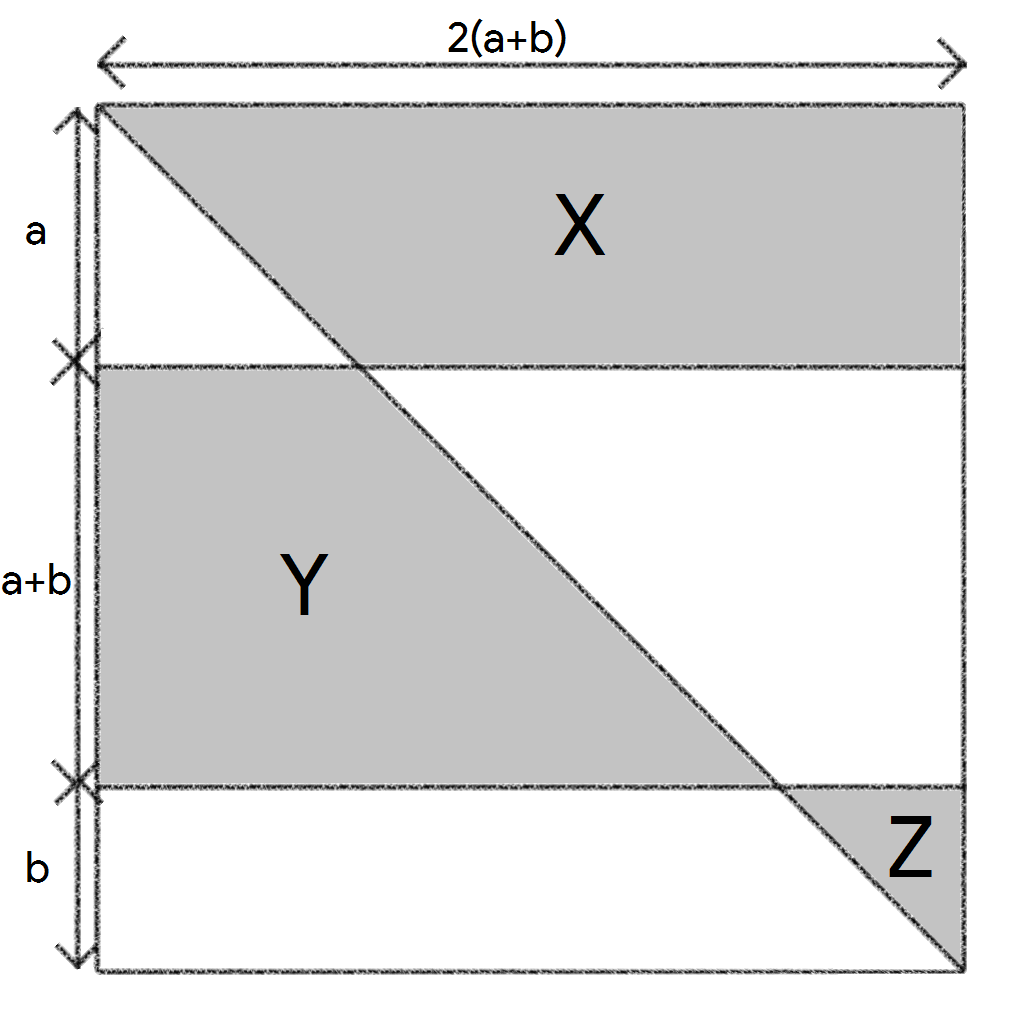# Areas All Sliced Up

Geometry Level 1Given that the above diagram is a square and the area of $Y=2016$, find the area of $X+Z$.

×